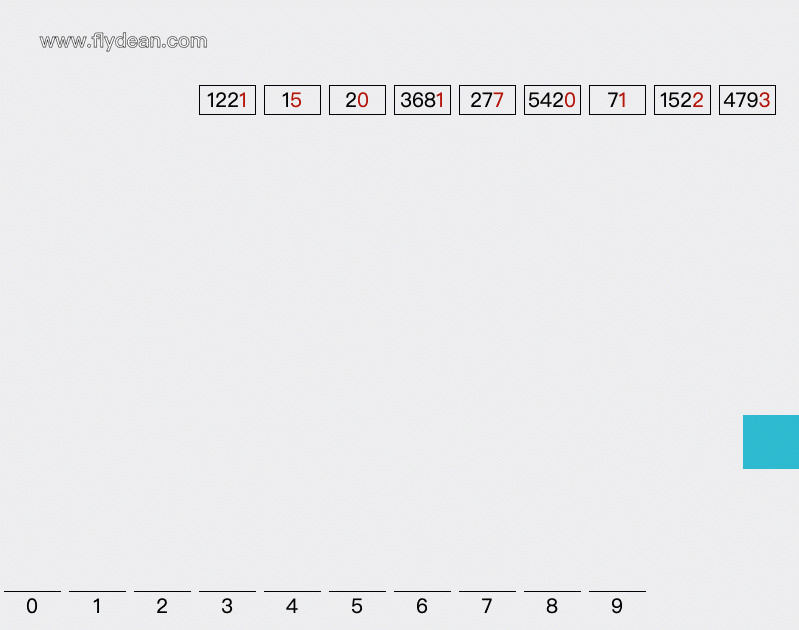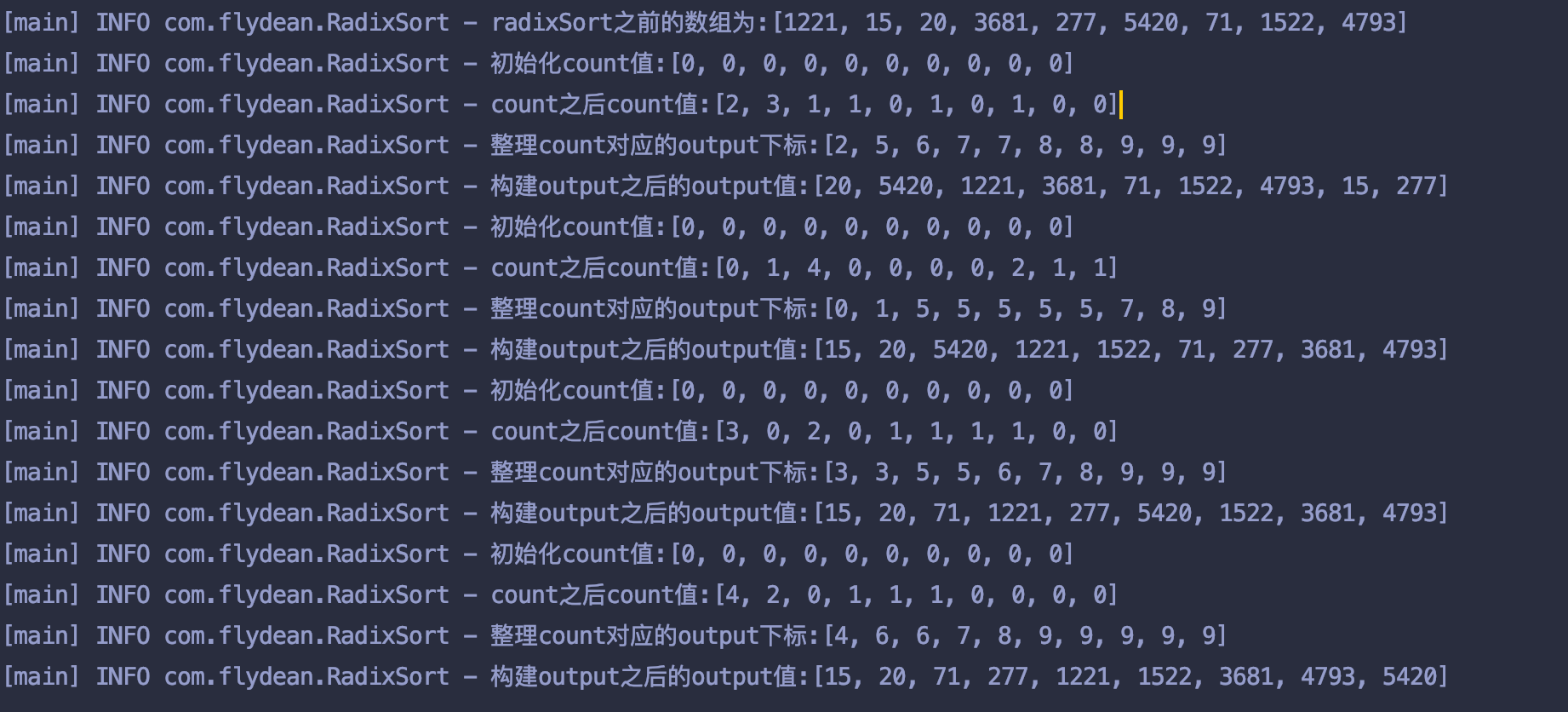09/27 09:43

# 基数排序的例子# 基数排序的java代码实现

doCountingSort方法除了传入数组外，还需要传入排序的位数digit，我们用1，10，100，1000来表示。

``````   public void doRadixSort(int[] array, int digit){
int n = array.length;

// 存储排序过后的数组
int output[] = new int[n];

// count数组，用来存储统计各个元素出现的次数
int count[] = new int;
Arrays.fill(count,0);
log.info("初始化count值:{}",count);

// 将原始数组中数据出现次数存入count数组
for (int i=0; i<n; ++i) {
count[(array[i]/digit)%10]++;
}
log.info("count之后count值:{}",count);

// 这里是一个小技巧，我们根据count中元素出现的次数计算对应元素第一次应该出现在output中的下标。
//这里的下标是从右往左数的
for (int i=1; i<10; i++) {
count[i] += count[i - 1];
}
log.info("整理count对应的output下标:{}",count);
// 根据count中的下标，构建排序后的数组
//插入一个之后，相应的count下标要减一
for (int i = n-1; i>=0; i--)
{
output[count[(array[i]/digit)%10]-1] = array[i];
count[(array[i]/digit)%10]--;
}
log.info("构建output之后的output值:{}",output);

//将排序后的数组写回原数组
for (int i = 0; i<n; ++i)
array[i] = output[i];
}
``````

``````public int getMax(int[] array)
{
int mx = array;
for (int i = 1; i < array.length; i++)
if (array[i] > mx){
mx = array[i];
}
return mx;
}
``````

``````    public static void main(String[] args) {
int[] array= {1221, 15, 20, 3681, 277, 5420, 71, 1522, 4793};
//拿到数组的最大值，用于计算digit
//根据位数，遍历进行count排序
for (int digit = 1; max/digit > 0; digit *= 10){
}
}
``````# 基数排序的时间复杂度

d是需要循环的轮数，也就是数组中最大数的位数。假如数组中最大的数字用K表示，那么d=logb(k)。

learn-algorithm

0
0 收藏

### 作者的其它热门文章0 评论
0 收藏
0